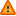FAQ/onesp - CBU statistics WikiYou should trust both wikis because the password could be read by the particular administrators.

Clear message
location: FAQ / onesp

# When and how do I evaluate a one-sided test and quote a one-sided 95% confidence interval

Steve Simon has written a very good article (see here) explaining how to evaluate one and two-sided p-values. if this link is broken the wording is reproduced here.

One-tailed tests provide more power to detect an effect, so it may be tempting to use a one-tailed test whenever you have a hypothesis about the direction of an effect. Before doing so, consider the consequences of missing an effect in the other direction. Imagine you have developed a new drug that you believe is an improvement over an existing drug. You wish to maximize your ability to detect the improvement, so you opt for a one-tailed test. In doing so, you fail to test for the possibility that the new drug is less effective than the existing drug. The consequences in this example are extreme, but they illustrate a danger of inappropriate use of a one-tailed test.

Another example where you would use a one-tailed test is for testing the discriminability of two sets of tests for diagnosing dementia. You could produce a 2x2 table of

For example

 Dementia_1 Normal_1 Dementia_2 a b Normal_2 c d

where _1 represents a gold standard reference test of dementia and _2 our own new quicker and simpler dementia diagnosis test. The tool is simpler and less time consuming to use that the standard diagnostic tool so would be advantageous to use clinically both for patients and clinicians.

We can assess the proportions sensitivity (a/(a+c)) and specificity (d/(b+d)) to ensure our new test ddoes a good job of identifying dementia cases whilst not giving too many false positive results.

Suppose we wish to test that sensitivity is at least 90%. We are not interested in sensitivities greater than 90% since our interest is in detecting unacceptable values of sensitivity which will be lower than 90%. In this case, therefore, we would carry out a one-tailed test (using, for example, a one-sample binomial test). In testing our new quicker tool we are only interested in testing if it is less effective than our existing diagnostic tool. In this scenario, a one-tailed test would be appropriate.

An associated question is:

How do we report a one-sided confidence interval?

This webpage looks at the case where we are testing the one-sided hypothesis mu0 > mu. It is easy to work out the analogous one-sided case mu0 < mu which we describe below.

For mu0 < mu we would actually use a 95% CI with 50% above the mean and 45% below it.

So 50% above the mean for a normal distribution is +infinity (or the maximum value possible) since this corresponds to the 100th percentile! The remaining 45% corresponds to the 5% cut-off which is 1.645 sds below the mean so the lower limit is mean - 1.645 x se (the analogous endpoint to the one described on the webpage).

This make sense as we are only interested in our hypothesis test of asking is our value lower than a particular value so only in effect estimate an endpoint at the lower end.